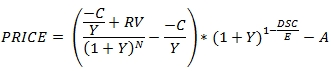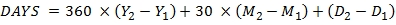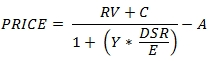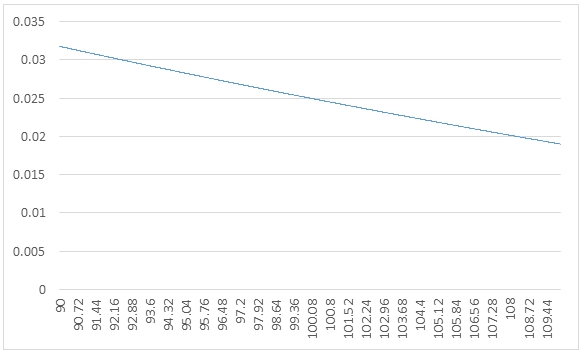## Calculating the price of a bond in SQL Server

Jul 1

Written by: Charles Flock
7/1/2014 2:36 PMWe look at some of the math behind the calculation of the price of a bond with regular periodic coupons, talk about how yield and accrued interest are calculated, and make some comparisons between the Excel functions and the XLeratorDB functions. We also talk about 2 new function that we have added to XLeratorDB that make it easier to see how PRICE and YIELD functions came up with their results.
The price equation(s).

The formula for calculating the price of a bond that pays regular periodic interest and has more than one period to redemption is:Where

 C = 100 * coupon rate / frequency Y = yield / frequency RV = redemption value DSC = number of days from settlement to coupon N = the number of coupons between the settlement date and the maturity date E = the number of days in the current coupon period A = C * accrued days / E

The real challenges in calculating the price of the bond isn't really the math (which is straightforward) but in assumptions made about the calendar. Let's look at how some of those play out. For purposes of this example, we will use the following data:
DECLARE @settlement as datetime = '2014-05-01'
DECLARE @maturity as datetime = '2034-06-15'
DECLARE @rate as float = 0.025
DECLARE @yield as float = .0276
DECLARE @redemption as float = 100
DECLARE @frequency as float = 2
DECLARE @basis as float = 1
The SQL to call the price function is as follows:
SELECT wct.PRICE(@settlement,@maturity,@rate,@yield,@redemption,@frequency,@basis)
The calculation of the following values is straightforward:
DECLARE @C as float = 100 * @rate / @frequency
DECLARE @Y as float = @yield / @frequency
DECLARE @RV as float = @redemption
Let's look at how the rest of the values are calculated starting with N.
N is the number of coupons payable from the settlement date through to the maturity date of the bond. Another way of saying this is that it's the number of coupon dates greater than the settlement date and less than or equal to the maturity date.
The COUPNUM function returns this value. But since the coupon dates are not passed into the PRICE function, it's worth spending a little bit talking about how the coupon dates are calculated. Coupon dates are calculated backwards from the maturity date of the bond, using the @frequency value. For example, if @frequency = 2 then coupons are paid twice-yearly or every six months. Thus you can calculate the number of coupons by counting backwards in six-month increments until the coupon date is less than or equal to the settlement date.
There's a little twist, however. In many systems, including Excel, if the maturity date is the last day of the month then all the coupon dates are assumed to occur on the last day of the month. There is no way of getting around this rule in Excel, even though there are certainly bonds issued with a maturity date of September 30th, for example, with coupons payable on the 30th of March and the 30th of September. In XLeratorDB, we have added more 'basis' codes which tell us whether to make the coupons always payable at the end of the month or to use the day of the month of the maturity date.
If we keep track of the coupon dates as we count backwards from the maturity date then in addition to knowing the value for N, we should also have the previous coupon date and the next coupon date (these are the values returned by the COUPPCD and COUPNCD functions).
The value for E is the number of days in the coupon period, i.e. the period defined by the previous coupon date and the next coupon date. When the basis code is 1 then the number of days is the actual number of days from the previous coupon date to the next coupon date. When the basis code is 0, 2, or 4, then the number of days is 360 / @Frequency. When the basis code is 3, then the number of days is 365 / @Frequency. This is the value returned by the COUPDAYS function.
The value for DSC is the number of days in the period (E) minus the number of days of accrued interest. This is the value returned by the COUPDAYSNC function and to understand how that works we need to look at how the accrued days are calculated.
The accrued interest is calculated as the number of accrued days divided by the number of days in the period multiplied by the coupon amount. For basis codes 1, 2, 3 (actual/actual, actual/360, and actual/365) the number of accrued days is the actual number of days from the previous coupon date to the settlement date. For basis codes 2 and 3 this means that it is possible for the number of accrued days to be greater than then number of days in the period.
For basis codes 0 and 4 the days are counted slightly differently. Excel defines basis code 0 as US (NASD) 30/360 and basis code 4 as European 30/360, though it doesn't actually explain what that means. The basic formula for these calculations is:For the US rule, you can think of all of values being derived in the following way:

 Y1 = YEAR(wct.COUPPCD(@settlement,@maturity,@frequency,0)) Y2 = YEAR(wct.COUPNCD(@settlement,@maturity,@frequency,0)) M1 = MONTH(wct.COUPPCD(@settlement,@maturity,@frequency,0)) M2 = MONTH(wct.COUPNCD(@settlement,@maturity,@frequency,0)) D1 = DAY(wct.COUPPCD(@settlement,@maturity,@frequency,0)) D2 = DAY(wct.COUPNCD(@settlement,@maturity,@frequency,0))

According to Standard Securities Calculation Methods: Fixed Income Securities Formulas for Price, Yield, and Accrued Interest (Volume 1, Third Edition) by Jan Mayle, you then apply the following adjustments:
(i)     If the security follows the end-of-month rule and D2 is the last day of February and D1 is the last day of February then change D2 to 30.
(ii)   If the security follows the end-of-month rule and D1 is the last day of February then change D1 to 30.

(iii)   If D2 is 31 and D1 is 30 or 31 then change D2 to 30.

(iv) If D1 is 31 then change D1 to 30.
For the European rule the initial Yi, Mi, and Di values are calculated as above and then modified in the following way according to 4.16(g) in the 2006 ISDA Definitions:
(i)     If D1 is 31 then change D1 to 30.
(ii)   If D2 is 31 then change D2 to 30.
It is much easier, however, to use the COUPDAYBS function which will automatically return the accrued days for you.
We are now ready to calculate all the inputs into the equation:
DECLARE @N as float = wct.COUPNUM(@settlement,@maturity,@frequency,@basis)
DECLARE @E as float = wct.COUPDAYS(@settlement,@maturity,@frequency,@basis)
DECLARE @DSC as float = wct.COUPDAYSNC(@settlement,@maturity,@frequency,@basis)
DECLARE @days_accrued as float = wct.COUPDAYBS(@settlement,@maturity,@frequency,@basis)
DECLARE @A as float = @C * @days_accrued / @E
This gives us all the inputs into our formula for more than one period to redemption, letting us run the following SQL.
SELECT
((-@C/@Y + @RV)/POWER(1+@Y,@N) -(-@C/@Y)) * POWER(1+@Y,1-@DSC/@E) - @A as PRICE
This produces the following result.
PRICE
----------------------
96.0043799057023

All this is by way of example to demonstrate the math behind the function. The best way to calculate the price is to just use the PRICE function.
SELECT
wct.PRICE(@settlement,@maturity,@rate,@yield,@redemption,@frequency,@basis) as PRICE
This produces the following result.
PRICE
----------------------
96.0043799057024
When the bond is settling in the final coupon period the calculation of the price is slightly different. A bond settles in the final coupon period when the settlement date is greater than or equal to the last coupon date prior to the maturity date of the bond. The formula in this case is:Where

 C = 100 * coupon rate / frequency Y = yield / frequency RV = redemption value DSR = number of days from settlement to redemption E = number of days in the current coupon period A = C * accrued days / E

As in the previous example, the best way to calculate the price of the bond is to use the XLeratorDB PRICE function, though it is possible to use other XLeratorDB functions and plug those values into the PRICE formula. The following SQL produces the result of both the function and formula.
DECLARE @settlement as datetime = '2014-05-01'
DECLARE @maturity as datetime = '2014-07-15'
DECLARE @rate as float = 0.0190
DECLARE @yield as float = .0005
DECLARE @redemption as float = 100
DECLARE @frequency as float = 2
DECLARE @basis as float = 0

DECLARE @C as float = 100 * @Rate / @frequency
DECLARE @Y as float = @yield / @frequency
DECLARE @RV as float = @redemption

DECLARE @E as float = wct.COUPDAYS(@settlement,@maturity,@frequency,@basis)
DECLARE @DSR as float = wct.COUPDAYSNC(@settlement,@maturity,@frequency,@basis)
DECLARE @days_accrued as float = wct.COUPDAYBS(@settlement,@maturity,@frequency,@basis)
DECLARE @A as float = @C * @days_accrued / @E

SELECT
wct.PRICE(@settlement,@maturity,@rate,@yield,@redemption,@frequency,@basis) as [PRICE function]
,(@RV + @C)/(1 + @Y * @DSR/@E) - @A as [PRICE formula]
This produces the following result.
PRICE function         PRICE formula
---------------------- ----------------------
100.380181205142       100.380181205142

In our environment we are able to process about 37,000 rows per second for the price calculation.

Differences between the XLeratorDB PRICE function and the Excel PRICE function
The XLeratorDB PRICE function contains all the functionality of the EXCEL PRICE function and has been exhaustively tested against the Excel function and there are no differences between the results produced in Excel and the result produced by XLeratorDB. However, the XLeratorDB version of the function provides support for the following features which are not supported in Excel:
·         Monthly and bi-month frequencies
·         More day-count conventions including 30E+/360, NL/360, NL/365, NL/actual, and Actual/364
·         Support for non-end-of-month date calculations for all day-count conventions
·         Negative yields
·         Negative interest rates

Calculating Yield
It's important to understand how the PRICE formula works but the fact of the matter is that most bonds trade on price and the yield is inferred from the price. For example, when the US Treasury auctions off bonds, it sets the maturity date and the coupon rate for the bond and then accepts bids which are quoted as a price (a somewhat different process is used for the auctioning of US Treasury bills, but that's a discussion for another time). The yield on those bonds is then calculated from the price. When US treasury bonds are traded in the secondary market they also trade on price.
The yield is related to the price because it is the value that discounts all the cash flows back to the price. Unless the bond is settling in the final coupon period, there is no closed-form solution for the yield. The only way to come up with a solution for yield is through iteration. While it is beyond the scope of this article to go into iteration theory, the XLeratorDB YIELD function does use the Newton-Raphson method to solve for the yield. The effectiveness of the Newton-Raphson technique is strongly influenced by the value of the initial guess and, in general, the XLeratorDB YIELD function finds a solution for yield in slightly less than 4 iterations. In our test harness this translates into a processing speed of about 18,000 rows per second for the yield calculation.
The function call for YIELD parallels the function call for PRICE with the exception that the yield value is passed into PRICE and the price value is passed into YIELD. Using the same type of format that we used to explain the price function, this is what calling the YIELD function in SQL looks like:
DECLARE @settlement as datetime = '2014-05-01'
DECLARE @maturity as datetime = '2034-06-15'
DECLARE @rate as float = 0.0250
DECLARE @price as float = 96.0043799057024
DECLARE @redemption as float = 100
DECLARE @frequency as float = 2
DECLARE @basis as float = 1

SELECT
wct.YIELD(@settlement,@maturity,@rate,@price,@redemption,@frequency,@basis) as YIELD
This produces the following result.
YIELD
----------------------
0.0276000000000131
You can always verify the accuracy of the YIELD calculation by running SQL that looks something like this.
SELECT
wct.PRICE(
@settlement
,@maturity
,@rate
,wct.YIELD(
@settlement
,@maturity
,@rate
,@price
,@redemption
,@frequency
,@basis
)
,@redemption
,@frequency
,@basis
) - @price
This produces the following result.

----------------------
-1.97388771994156E-11
and in almost all cases the absolute value of the result will be less than .00000001
While you occasionally might want to calculate the yield for a single bond, the advantage to having the yield calculation in SQL Server is that it gives us the ability to calculate the yield on thousands of rows with a single T-SQL statement.
Let's run the following SQL which demonstrates the relation between price and yield.
SELECT
k.SeriesValue as PRICE
,wct.YIELD(
@settlement
,@maturity
,@rate
,k.SeriesValue
,@redemption
,@frequency
,@basis
) as YIELD
FROM
wct.SeriesFloat(90,110,.01,NULL,NULL)k

This statement quite easily returns 2,001 rows showing the yield for each price between 90 and 110 in increments of .01. We can copy the resultant table into Excel to produce the following graph.This graph demonstrates the inverse relationship between price and yield: as price goes up yield goes down.
The yield for bonds which settle in the final coupon period can be solved in a closed-form and is covered in the XLeratorDB documentation for the YIELD function.

Differences between the XLeratorDB YIELD function and the Excel YIELD function
As with the PRICE function the XLeratorDB YIELD function contains all the functionality of the EXCEL YIELD function and has been exhaustively tested against the Excel function. There are some differences between the two functions, which we believe are erroneous results returned by the Excel function and which we have documented here.

Additionally, the XLeratorDB version of the function provides support for the following features which are not supported in Excel:
·         Monthly and bi-month frequencies
·         More day-count conventions including 30E+/360, NL/360, NL/365, NL/actual, and Actual/364
·         Support for non-end-of-month date calculations for all day-count conventions
·         Negative yields
·         Negative interest rates

Accrued Interest calculations
Accrued interest is one of the components of the PRICE calculation. In fact, it is easy to think of the PRICE calculation as the discounted cash flow value of the coupons and the redemption amount minus the accrued interest. Getting the accrued interest right is an important part of the equation.
Rather than replicate the Excel ACCRINT function, we developed a function named BONDINT which calculates the accrued interest using the same data as the PRICE and YIELD functions, which makes it pretty straightforward to map data used in the PRICE function to the data in the BONDINT function. The Excel function actually uses different data and produces unexpected results which we have documented here.
The following SQL summarizes the math behind the accrued interest calculation.
DECLARE @settlement as datetime = '2014-05-01'
DECLARE @maturity as datetime = '2014-07-15'
DECLARE @rate as float = 0.0190
DECLARE @frequency as float = 2
DECLARE @basis as float = 0

DECLARE @C as float = 100 * @Rate / @frequency

DECLARE @E as float = wct.COUPDAYS(@settlement,@maturity,@frequency,@basis)
DECLARE @days_accrued as float = wct.COUPDAYBS(@settlement,@maturity,@frequency,@basis)
DECLARE @A as float = @C * @days_accrued / @E

SELECT
wct.BONDINT(@settlement,@maturity,@rate,100,@frequency,@basis) as [BONDINT function]
,@C * @days_accrued / @E as [BONDINT formula]

This produces the following result.
BONDINT function       BONDINT formula
---------------------- ----------------------
0.559444444444444      0.559444444444444

As with the price function, the calculation of the accrued interest is the coupon amount multiplied by the ratio of the accrued days to the number of days in the period. As discussed above, the number of days in the period for basis codes 0, 2, and 4 is 360 divided by the frequency. For basis code 3 it is 365 divided by the frequency, and for basis code 1 it is the actual number of days from the previous coupon date to the next coupon date.
The accrued number of days for basis codes 1, 2, and 3 is the actual number of days from the previous coupon date to the settlement date. For basis codes 0 and 4 the rules for calculating the number of days are as described above.
In the PRICE function, the par value is always assumed to be 100. In the BONDINT function you have the ability to pass in the par value.

2 New functions
As you can see the PRICE, YIELD, and BONDINT functions calculate a variety of values (the previous coupon date, the next coupon date, the accrued days, the number of days in the coupon period, the number of days until the next coupon period) which might be of interest. There are a variety of functions in Excel (and thus a variety of functions in XLeratorDB) that can provide you with those values. However, it is far more efficient to calculate everything at once and return all values in a table-valued function. In XLeratorDB/financial 1.13 we have added the RPIFACTORS function which decomposes all the underlying values used in the PRICE or the YIELD calculation and returns the results to you in a single function call. The function call is very similar to the function call for the PRICE and YIELD function, with the exception that price and/or yield can be supplied to the function. If yield is entered, then the price is calculated (regardless of whether or not it was entered). If yield is NULL and price is supplied to the function then the yield is calculated.
Here's what the function looks like.
DECLARE @settlement as datetime = '2014-05-01'
DECLARE @maturity as datetime = '2034-06-15'
DECLARE @rate as float = 0.0250
DECLARE @yield as float = .0276
DECLARE @price as float
DECLARE @redemption as float = 100
DECLARE @frequency as float = 2
DECLARE @basis as float = 1

SELECT
*
FROM
wct.RPIFACTORS(
@settlement
,@maturity
,@rate
,@price
,@yield
,@redemption
,@frequency
,@basis
)
This produces the following result, which has been reformatted to make it easier to read.

 PrevCoup NextCoup A DSC E N C P AI Y 12/15/2013 6/15/2014 137 45 182 41 1.25 96.00437991 0.940934066 0.0276

We can see that we have the results of all the underlying calculations contained in the output of the table-valued function as well as calculation of the price and the yield. This greatly simplifies figuring out the underlying math.
Second, since we have an easy, efficient way to calculate the underlying factors we thought that it made sense to provide a function that would return the price without doing any of the underlying calculations. In other words, there are no dates to deal with.
The RPI function takes all the underlying components on the PRICE or YIELD calculation and returns the price or yield based on those factors. For example, using the example from above, we could calculate the price with the following SQL.
SELECT
wct.RPI(
137,     --@A
45,      --@DSC
182,     --@E
41,      --@N
.025,    --@R
0.0276, --@Y
NULL,    --@P
2,       --@F
100      --@RV
) as PRICE
This produces the following result.
PRICE
----------------------
96.0043799057024

Calculating bond prices and yield in SQL Server makes a great deal of sense if you are storing your bond and security master data on your SQL Server database. XLeratorDB provides a highly-optimized, efficient, scalable solution to support all of your calculation requirements. XLeratorDB has more than 2 dozen functions specifically for bond figuration including calculations for convexity, duration, modified duration, bonds with odd first and/or last periods, and stepped coupon bonds. Additionally, using SQL Server as the platform for your bond calculations provides you with the world's most flexible and widely supported tool for managing data; SQL.

Dow
nload the 15-day free trial and discover how quickly you can be solving your bond figuration problems.

Tags:
Categories:
Location: BlogsThe WestClinTech Blog

## Search Blogs

 KeywordsPhrase

## Blog Archives

Archive
 < September 2023 >
SunMonTueWedThuFriSat
272829303112
3456789
10111213141516
17181920212223
24252627282930
1234567
Monthly
Go

### SupportCopyright 2008-2023 Westclintech LLC         Privacy Policy        Terms of Service